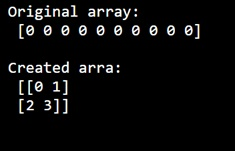# How to use numpy.arange() with pandas Series?

Learn, how to use numpy.arange() with pandas Series?
Submitted by Pranit Sharma, on December 31, 2022

NumPy is an abbreviated form of Numerical Python. It is used for different types of scientific operations in python. Numpy is a vast library in python which is used for almost every kind of scientific or mathematical operation. It is itself an array which is a collection of various methods and functions for processing the arrays.

## Using numpy.arange() with pandas Series

There are three types of arguments that can be passed all together or some of them alone like (stop), (start, stop), (start, stop, step).

If we pass an integer argument, this method works similar to the range function.

Syntax:

```numpy.arange(
[start, ]stop,
[step, ]dtype=None,
*,
like=None
)
```

Parameter(s):

• startinteger or real, optional: Start of interval. The interval includes this value. The default start value is 0.
• stopinteger or real: End of interval. The interval does not include this value, except in some cases where the step is not an integer and floating point round-off affects the length of out.
• stepinteger or real, optional: Spacing between values. For any output out, this is the distance between two adjacent values, out[i+1] - out[i]. The default step size is 1. If the step is specified as a position argument, a start must also be given.
• dtypedtype, optional: The type of the output array. If type is not given, infer the data type from the other input arguments.

Let us understand with the help of an example,

## Python code to use numpy.arange() with pandas Series

```# Import numpy
import numpy as np

# Import pandas
import pandas as pd

# Creating an array with arrange method
arr = np.arange(0, 5, 0.5, dtype=int)

# Display original array
print("Original array:\n",arr,"\n")

# Creating an array with arrange method
arr  = np.arange(4).reshape(2, 2)

# Display array
print("Created array:\n",arr,"\n")
```

Output: